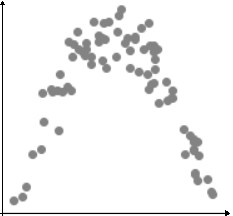# The Pearson's correlation

Modified on Mon, 30 Aug 2021 at 09:14 AM

# Definition

The correlation is a measure of concordance in variation between two variables. The Pearson’s correlation coefficient r measures the intensity and the direction of the correlation between two variables in case of the presence of a linear relationship between them.

It varies between -1 and +1. The sign of the relation indicates the direction of the correlation: it could be positive or negative. An interpretation of the intensity of the correlation has been proposed by Evans (1996) :

• < 0.2: very weak
• .20-.39: weak
• .40-.59: moderate
• 60-.79: strong
• > 0.8: very strong

To perform a Pearson’s correlation, the variables analysed have to follow a normal distribution, to have a supposed linear relationship and to be measured on an interval scale (continuous).

# In practice

The correlation assessment helps to evaluate the degree of association between two variables, i.e. to indicate how the variables evolve together: do they evolve together ? If yes, do they evolve together in the same direction and how much ?

For example, in medical research we usually can suppose that the age is positively correlated with the disease risk, meaning that when the age improve, the risk of diseases improve. We should thus expect a correlation coefficient between "age" and "disease risk" be: r > 0.

We can also suppose that the age is negatively correlated with the muscular force after 40 years old, meaning that when the age improve, the muscular force decrease. We should thus expect a correlation coefficient between "age" and "muscular force" be: r < 0.

# Warning

The Pearson correlation test is efficient to highlight a correlation between variables only if the relationship between them is linear. In the case shown below, the correlation coefficient of Pearson will not detect any correlation between the two variables even if there are obviously correlated.# How to use it on EasyMedStat ?

1. Go to Statistics > Test variables
2. Select two Numeric variable you would like to analyze and click on "Which tests can I do ?"
3. Click on "Find a numeric correlation between ... and ..."

If the assumptions of the Pearson correlation are not met, Spearman's correlation will be calculated instead.